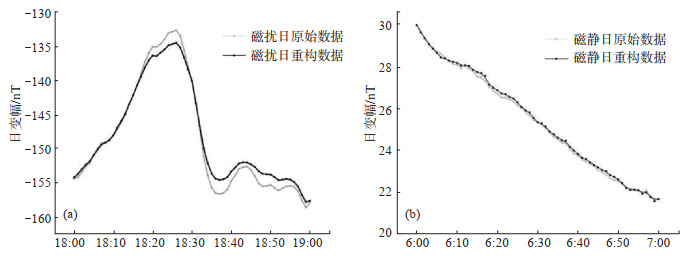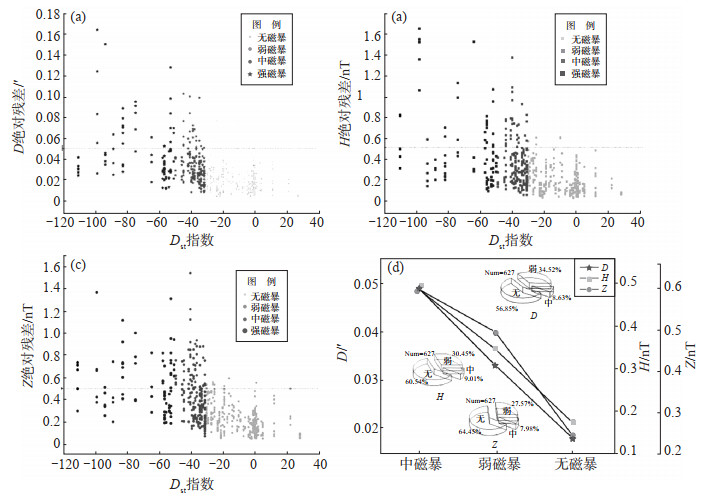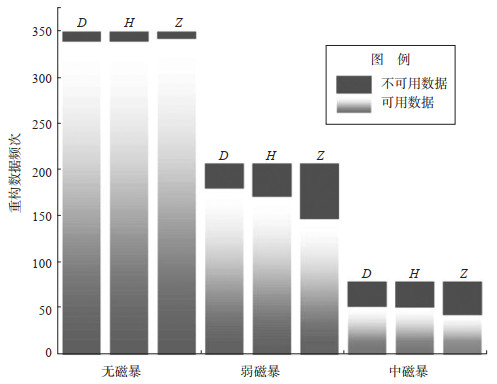﻿ 基于空间加权法的地磁数据重构精度与<i>D</i><sub>st</sub>指数的关系文章快速检索
 地震地磁观测与研究2020, Vol. 41Issue (3): 91-97  DOI: 10.3969/j.issn.1003-3246.2020.03.0120

### 引用本文GU Huidong, YAO Xiuyi, LI Donglin. Study on the relationship between the reconstruction accuracy of geomagnetic data based on spatial weighting method and Dst index[J]. Seismological and Geomagnetic Observation and Research, 2020, 41(3): 91-97. DOI: 10.3969/j.issn.1003-3246.2020.03.012.### 文章历史

1. 中国云南 674100 丽江地震台;
2. 中国昆明 650224 云南省地震局;
3. 中国昆明 650224 云南大学

Study on the relationship between the reconstruction accuracy of geomagnetic data based on spatial weighting method and Dst index
GU Huidong 1, YAO Xiuyi 2, LI Donglin 3
1. Lijiang Seismic Station, Yunnan Province 674100, China;
2. Yunnan Earthquake Agency, Kunming 650224, China;
3. Yunnan University, Kunming 650224, China
Abstract: In order to study the influence of magnetic activity level on the geomagnetic data reconstruction, this paper took minute sampling data at 11 geomagnetic stations in Yunnan Province as a research object to reconstruct geomagnetic observations by using the spatial weighting method and analyze the relationship between the accuracy of reconstructed data and the geomagnetic activity index (Dst). The results show that the accuracy of reconstructed geomagnetic data is negatively correlated with the absolute value of Dst index. When the Dst index is greater than -30 (no magnetic storm), the D-component reconstructed data with an absolute error of less than 0.05 points accounts for 97.1%. The proportion of the H and Z components reconstructed data with an absolute error of less than 0.5 nT is 97.1% and 97.9%, respectively, indicating a high reconstruction accuracy. When weak or moderate magnetic storms occur, the absolute errors of the reconstructed data for D, H, and Z components increase with the increase of the absolute value of the Dst index, giving a low reconstruction accuracy. The results in this paper can provide references for the reconstruction of geomagnetic data due to abnormal observations or missing records.
Key words: geomagnetic data    spatial weighting method    data reconstruction    Dst index
0 引言

1 数据重构原理与方法

1.1 数据重构原理

 ${{y}_{ij}}=\frac{{{B}_{j}}-{{B}_{\min }}}{{{B}_{\max }}-{{B}_{\min }}}$ (1)

 $\sum\limits_{i=1}^{N}{{{W}_{i}}}=1$ (2)
 ${{W}_{1}}\cdot {{R}_{1}}={{W}_{2}}\cdot {{R}_{2}}=\ldots ={{W}_{N}}\cdot {{R}_{N}}$ (3)
 ${{Y}_{j}}=\sum\limits_{i=1}^{N}{{{W}_{i}}\cdot {{X}_{ij}}+r}$ (4)

1.2 台阶改正图 1 线性台阶改正结果 Fig.1 The result of liner-step correction

 ${{B}_{j}}^{\prime }={{B}_{j}}+\frac{\text{d}{{B}_{2}}-\text{d}{{B}_{1}}}{M}\times j-\text{d}{{B}_{1}}\ \ \ \ \ j=1, 2, 3, \ldots, M$ (5)

2 数据重构精度与Dst指数相关性分析 2.1 地磁空间相关性表 1 台站间地磁分量相关系数统计 Table 1 Statistics of correlation coefficients of geomagnetic observations between stations
2.2 计算结果

 $e=\frac{\sum{\left| f\left(n \right)-\hat{f}\left(n \right) \right|}}{N}\ \ \ \ \ \ n=1, 2, 3, \ldots$ (6)图 2 磁静、扰日重构关系 (a)磁扰日；(b)磁静日 Fig.2 The reconstructed and observed data for disturbed and quiet days图 3 重构数据相关分析 (a)Dst指数与D的绝对残差关系；(b)Dst指数与H的绝对残差关系；(c)Dst指数与Z的绝对残差关系；(d)Dst指数与残差的线性相关性 Fig.3 Correlation analysis of the reconstructed data
2.3 结果分析图 4 磁场活动水平与可用、不可用数据统计关系 Fig.4 The statistical relationship between magnetic field activity levels and data availabilities

3 结论与讨论

（1）磁扰日重构数据残差高于磁静日。

（2）随着Dst指数绝对值的增大，数据重构残差增大，重构精度减小，总体呈线性关系。

（3）无磁暴发生时，小于0.5 nT的DHZ三分量重构数据与原始数据的绝对残差值占比均在97%以上，重构后的数据可信度较高，重构精度较高。当发生弱磁暴、中等磁暴时，DHZ三分量重构数据与原始数据的绝对残差值随着Dst指数绝对值的增大而增大，重构精度减小。

 冯志生, 梅卫萍, 张苏平, 等. 2005. FHD磁力仪Z分量分钟值日变化空间相关性的初步应用[J]. 华南地震, 25(3): 1-7. DOI:10.3969/j.issn.1001-8662.2005.03.001 胡秀娟, 杨冬梅, 王静, 等. 2014. 磁通门磁力仪GM4定向方法的研究[J]. 震灾防御技术, 9(Z1): 609-614. 胡星星, 滕云田, 谢凡, 等. 2010. 磁通门磁力仪背景磁场的自动补偿设计[J]. 仪器仪表学报, 31(4): 956-960. 刘振兴. 2005. 太空物理学[M]. 哈尔滨: 哈尔滨工业大学出版社. 沈红会, 周加新, 张秀霞, 等. 2005. 直流输电对江苏地磁观测的影响分析[J]. 地震地磁观测与研究, 26(5): 65-70. DOI:10.3969/j.issn.1003-3246.2005.05.010 王仁明, 齐玮, 李冰.基于Dst指数的磁暴发生率统计分析[C]//国家安全地球物理丛书(七)——地球物理与核探测.北京: 中国地球物理学会, 2011: 318-322. 熊仲华, 刘运生. 1997. 地磁观测技术[M]. 北京: 地震出版社, 8-53. 徐文耀. 2003. 地磁学[M]. 北京: 地震出版社. 徐文耀. 2009. 地球电磁现象物理学[M]. 合肥: 中国科学技术大学出版社. 姚休义.地磁台站观测异常识别与数据重构技术研究[D].北京: 中国地震局地球物理研究所, 2015: 47-54. 姚休义, 滕云田, 杨冬梅, 等. 2016. 地磁观测数据重构技术研究[J]. 地震学报, 38(6): 878-888. 袁桂平, 李鸿宇, 张贵霞, 等. 2018. 地磁垂直分量Z日变幅逐日比及其与磁暴和地震的关系[J]. 地震, 38(1): 139-146. 张建国, 乔子云, 吕凤章, 等. 2007. 河北省地磁场变化规律研究[J]. 地震地磁观测与研究, 28(3): 8-12. DOI:10.3969/j.issn.1003-3246.2007.03.002 赵旭东, 何宇飞, 陈俊, 等. 2019. 基于地磁台站数据对磁暴期间环电流和场向电流的分布特征研究[J]. 地球物理学报, 62(9): 3209-3222. 朱兆才. 1989. 空间相关性分析在地磁观测研究中的应用[J]. 地震地磁观测与研究, 10(5): 44-49.